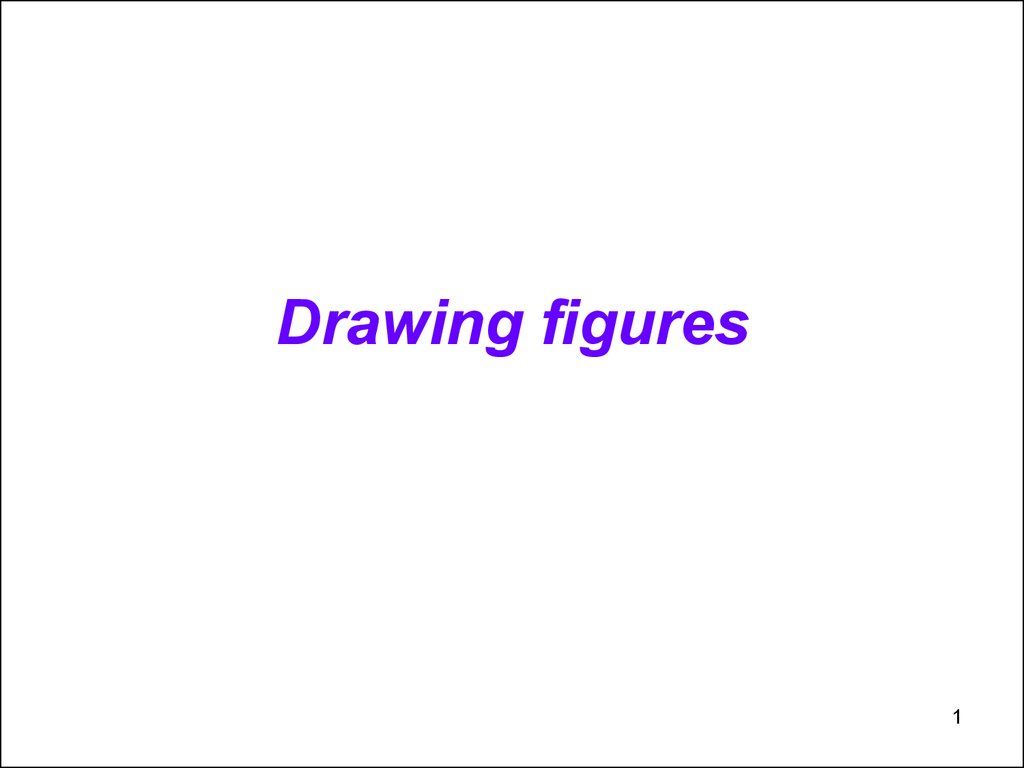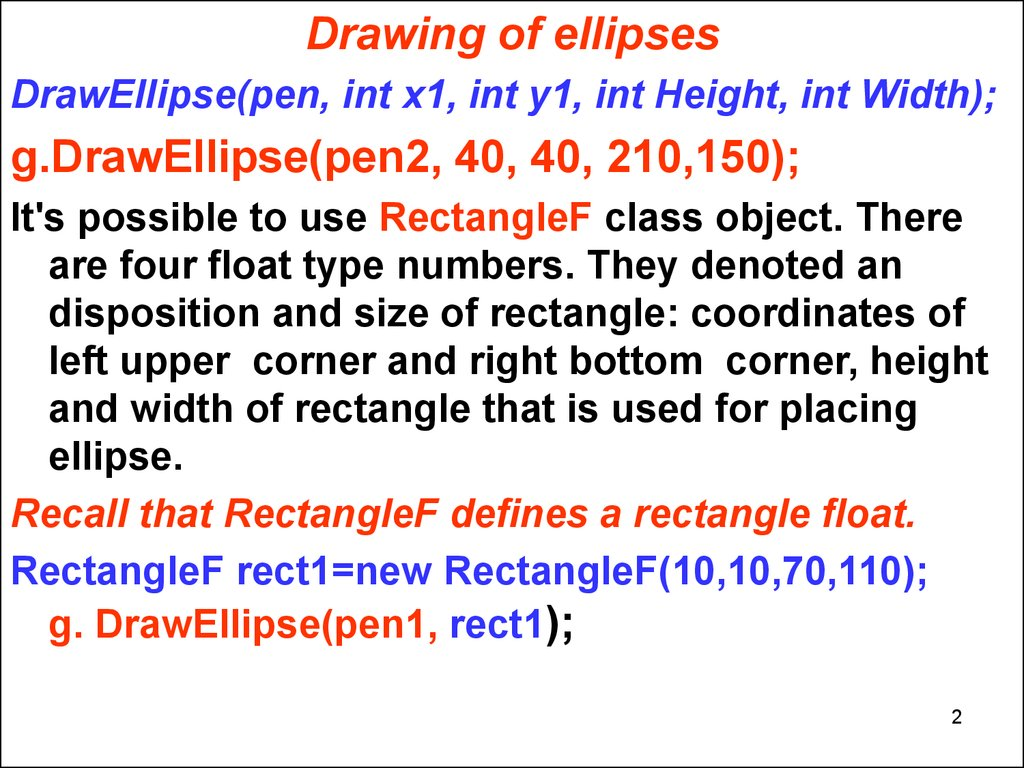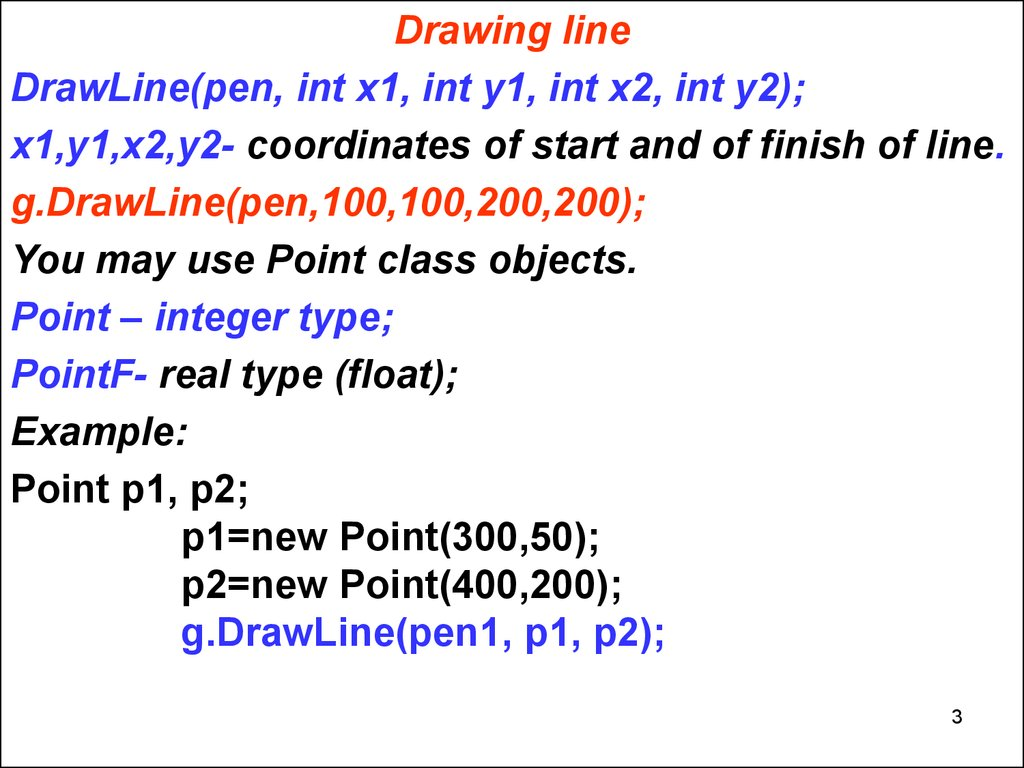# Lecture 10. Drawing figures C#

1

## 2.

Drawing of ellipses
DrawEllipse(pen, int x1, int y1, int Height, int Width);
g.DrawEllipse(pen2, 40, 40, 210,150);
It's possible to use RectangleF class object. There
are four float type numbers. They denoted an
disposition and size of rectangle: coordinates of
left upper corner and right bottom corner, height
and width of rectangle that is used for placing
ellipse.
Recall that RectangleF defines a rectangle float.
RectangleF rect1=new RectangleF(10,10,70,110);
g. DrawEllipse(pen1, rect1);
2

## 3.

Drawing line
DrawLine(pen, int x1, int y1, int x2, int y2);
x1,y1,x2,y2- coordinates of start and of finish of line.
g.DrawLine(pen,100,100,200,200);
You may use Point class objects.
Point – integer type;
PointF- real type (float);
Example:
Point p1, p2;
p1=new Point(300,50);
p2=new Point(400,200);
g.DrawLine(pen1, p1, p2);
3

## 4.

Drawing of curves
1) Bezier curve. This is smooth curve that is placed
close to four preset points.
DrawBezier(pen, p1,p2,p3,p4);
Example:
double[ ] q=new double;
Point[ ] w=new Point ;
for(int i=0;i<f2.Length;i++)
{
q[i]=100*Math.Exp(-i*0.1);
w[i].X=200+ (int) (i*2.5);
w[i].Y=150+ (int) q[i];
}
g.DrawBezier(pen,w,w,w,w);
4

## 5.

2) DrawCurve(pen, array_of_points);- the
smooth curve that passes through points
array.
Example:
PointF [ ] f= new PointF;
f.X=10;f.Y=100;
f.X=50; f.Y=150;
f.X=100;f.Y=240;
f.X=150; f.Y=130;
f.X=200;f.Y=50;
f.X=300; f.Y=10;
g.DrawCurve(pen, f );
5

## 6.

3) DrawClosedCurve(pen, array_of_points);-closed
smooth curve that passes through points array.
Example,
g.DrawClosedCurve(pen, f );
4) DrawPoligon(pen, array_of_points);-creates a
polygon by linking the points by direct lines.
Point [ ] p= new Point;
p.X=110;
p.Y=110;
p.X=230;
p.Y=130;
p.X=290;
p.Y=190;
g.DrawPolygon(pen2, p );
6

## 7.

Drawing of Arc
5) DrawArc(pen, int x, int y, int Height, int Width,
int Start_Angle, int End_Angle);- the arc is a part of
ellipse that is placed in rectangle with preset
Height and Width.
Start_Angle, End_Angle- these are start angle and
finish angle in degree;
g.DrawArc(pen, 200, 200, 100, 100, 10, 160);
6) Pie.
It differs from an arc because in this case arc's
ends are connected with center by radiuses.
DrawPie(pen, rect, StartAngle, EndAngle);
rect - this is an object of Rectangle or RectangleF
classes. It's possible merely to preset the
coordinates, height and width of rectangle.
g.DrawPie(pen2,100,100,350,350,0,280);
7